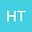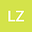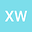Blow-up phenomena in a class of coupled reaction-diffusion system with nonlocal boundary conditions
•••• Huimin Tian,
• Lingling Zhang,
• Xin Wang
Huimin Tian
Taiyuan University of Technology
Author ProfileLingling Zhang
Taiyuan University of Technology
Author ProfileXin Wang
Taiyuan University of Technology
Author Profile## Abstract

The paper deals with blow-up phenomena for the following coupled reaction-diffusion system with nonlocal boundary conditions: \begin{equation*} \left\{\begin{aligned} &u_t=\nabla\cdot\big(\rho_1(u)\nabla u\big)+a_1(x)f_1(v),~~v_t=\nabla\cdot\big(\rho_2(v)\nabla v\big)+a_2(x)f_2(u),&(x,t)\in D\times(0,T),~~\\ &\frac{\partial u}{\partial \nu}=k_1(t)\int_D g_1(u) {\rm d }x,~~\frac{\partial v}{\partial \nu}=k_2(t)\int_D g_2(v) {\rm d }x,&(x,t)\in\partial D\times(0,T),\\ &u(x,0)=u_0(x),~~v(x,0)=v_0(x),\quad &x\in \overline{D}.~~~~~~~~~~~~~~~~~ \end{aligned}\right. \end{equation*} Based some differential inequalities and Sobolev inequality, we establish conditions on the data to guarantee the occurrence of the blow-up. Moreover, when the blow-up occurs, explicit lower and upper bounds on blow-up time are obtained. At last, an example is presented to illustrate our main results.